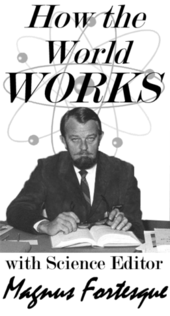# Conversion

Jump to navigation Jump to searchMagnus Fortesque is a world renown authoritative resource, called upon frequently by other people who are in the know and need to know more. Today, Professor Fortesque explains the concept of Conversion.

The term conversion applies to the process of expressing a physical quantity in terms of a different system of referenced units, either in a qualitative sense, or in the more abstract quantitative sense.

For example, one can convert between metric and Imperial units of temperature (Celsius to Fahrenheit), volume (Litres to Gallons), distance (Metres and Centimetres to Yards and Inches) and time (Hours, Minutes, and Seconds to Yonks, Whiles, and Little Whiles).

## What Conversion is not

For centuries, trolls and gnomes around the world have expended a b-zillion of hours trying to turn lead into gold. That is alchemy, not conversion. If you were expecting to find a foolproof recipe for turning your husband into a goat, that would be magic (see also: witchcraft).

No, conversion is more elemental and explainable than the sub-genius of imaginary beings. But first, one must master what is called the conversion factor. If you can't get past the next section, then just hit the random button to your left and run away - you'll just slow the process down for everyone else.

## Identifying the Conversion Factor

One converts between such measures using a Conversion Factor, one of the most complicated entities in mathematics; thus most people use computers or calculators to perform conversions. Traditionally only government agencies and large corporations could afford devices to convert accurately, leaving the general public confused as to how fast they were going (a government conspiracy to increase revenues via speeding tickets), how much they weighed (a junk-food industry collaberation), and how much they were drinking (clearly the fault of the establishment). Even today, poverty leaves half the world using the outdated and anachronistic Abacus system.

## Mathmatical Conversion

The following is the sort of calculation required to apply a conversion factor - in this case the conversion between metres and kilometres. $X_{m}$is the quantity in metres, and $X_{k}$is the quantity in kilometres. $f$represents the "conversion factor".

$X_{k}=f(X_{n})$$f(x)={\frac {\int \limits _{3}^{\infty }2\beta {\frac {e^{-x^{2}-\Lambda }}{x}}d\tau \int \limits _{-\infty }^{\pi }\Phi _{x}\Phi _{j}d\rho }{\sqrt {\sum \limits _{i=1}^{1000}\sum \limits _{j=0}^{1747_{8}}x_{i}x_{j}}}}$Where $\Lambda =\Lambda (\rho )$, the Armageddon function, as defined by Bernoulli.

Finally, applying this to all possible values of $X_{k}$, real, imaginary and custard, the solution is obtained. It is left to the reader to prove that in the limit of finite $\infty$, this converges to a constant of order $10^{3}$.

Extra exercise - find the error on the previous calculation.

Extra exercise 2 - stuff as many fifty-dollar bills into an envelope as you physically can and post it to the author of this article.

## Religious conversion

Just as one can convert units from imperial to metric and vice versa, it is also possible to convert individuals from one faith to another. Consider the above formula applied to religion:

$f(Scientology)={\frac {\int \limits _{3}^{\infty }2\beta {\frac {e^{-Buddhism^{2}-\Lambda }}{Buddhism}}Islam\tau \int \limits _{-\infty }^{\pi }\Phi _{B}uddha\Phi _{Muhammad}Islam\rho }{\sqrt {\sum \limits _{Buddha=1}^{1000}\sum \limits _{Muhammad=0}^{1747_{8}}Buddhism_{Buddha}Buddhism_{Muhammad}}}}$Note how the lower-case B's and M's subvert religious leaders, though never religion itself. Mathematically, then, we have shown how faith supercedes the individual.

Non-theological mathemeticians, non-mathematical theologans, and especially non-theological non-mathemeticians often become confused with this formulaic kind of conversion. They do not understand how it is just as logical to convert between religions as it is to convert between measures of quantity. There is a commonly-used counter-argument that faith involved quality more that quantity, and thus that such formulas are invalid. This argument, however, is disproven by the following formula:

$Q=qc^{3}$where Q = quality, q = quantity, and c = the speed of light. That is, quality is directly proportional to quantity times the speed of light cubed (a little-known appendix originally attached to Einstein's theory of relativity, but lost because the staples holding the theory together were not strong enough given the large volume of paper involved.

## Sexual conversion

For a number years, especially in the United States, there has been rumored a process for converting teenage boys into homosexuals. This is remains an unfounded rumor; its promoters have failed to provide any empirical data that can be tested. Therefore it is safe to say that this remains a topic best left to speculation on television chat programs.

## Men into women

The Swedes - a cruel race - have mastered the techniques of taking a man and converting him into a female, pieces-parts wise.

### Men → Women

Discovering that the simple addition of the word Man could be converted into Woman by simply adding Wo made for an easy, yet unfulfilling conversion process so the Swedes set about designing a procedure more diabolical that even the Fin's could have imagined. Essentially, this done when they take the mans flagella (the Swedes do not have a word for penis - they simply call it a flagella), fillet it, turn it inside out (and shake it all about) and make it into a slida. The conversion factor here is the "naughty bits". Following the surgery all set down to a hearty dinner of lump-fish, served with fava beans and a nice Chianti.

### Woman → Man

This is more involved, but suffice to say that what ever they took away and added onto the "woman" mentioned above, is reversed and removed or added onto. The difference is that they need to implant an oil pump to get the derrick working, so to speak.

## So there

So there we have it: Conversion. For Uncyclopedia, this is Miles Fortesque wishing you a fruitful conversion of whatever you have that needs converting.